|

# 餐厅设计实用主义者是怎样考虑的?

餐厅，算是家中最有人情味儿也是最热闹的地方，倘若没有它，夸张点说只能叫酒店、客栈、旅馆……但有了它，家便有了该有的温度。如此重要的空间却往往只是见缝插针地安排一个餐桌了事。

那么一个实用主义者到底该如何考虑和设计餐厅呢？

1.大户型餐厅

一般来说200平方米以上的户型常配置18平方米以上的餐厅，它可以独立成一个空间，这时我们该配备大尺寸的家具，如果过小会让空间略显空洞。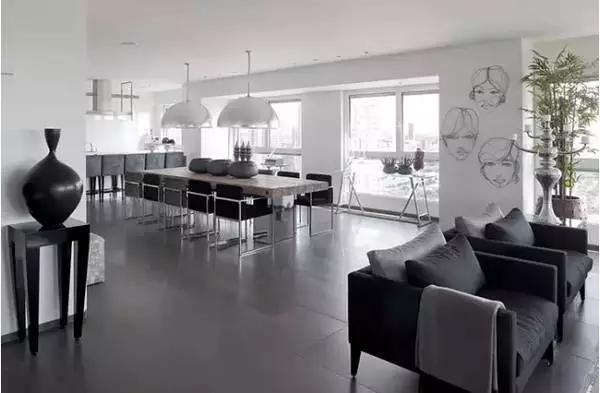这样的餐厅适用10人座长餐桌。10人以上的长餐桌最能凸显大气，而且占据整个空间，让餐厅更加充实。当然，餐桌样式和饮食习惯也有关系，国人更喜欢圆形餐桌。无论是哪种餐桌，想要占据面积大的餐厅，必须有足够的分量。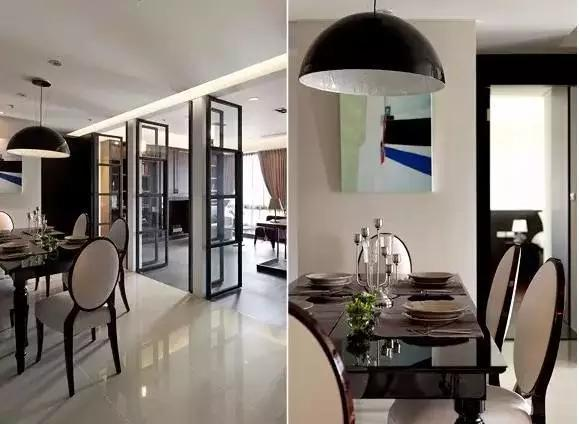餐柜一定要高。与6-12平方米的空间相反，大面积的餐厅必须要有足够高度的餐柜和餐椅，这样才不至于让人觉得空间过于空洞。此外，餐椅的椅背可以稍高，从垂直空间上填充大空间。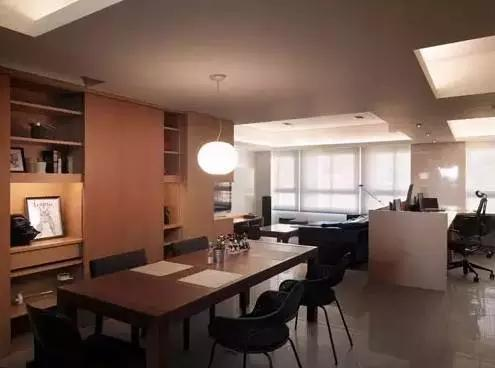2.一般户型餐厅

一般150平方米以上的户型常配置6-12平方米的餐厅，这样的餐厅说大不大，说小也不小，除了能容纳一张4-6人的餐桌外，还能加入餐柜。餐柜高度要适中。虽说可以在6-12平方米的餐厅内加入餐柜，但餐柜的高度也不能太高，只要比餐桌高一点就行，不超过82cm为原则，这样不会给空间造成压迫感。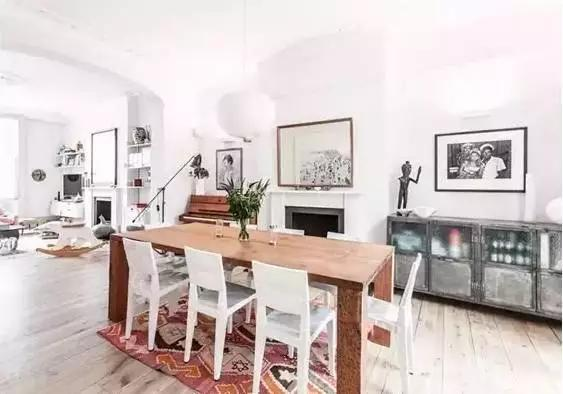餐椅的椅背不超过90cm为宜。除了餐柜的高度要适中外，餐桌和餐椅的高度也需要注意，90cm长度的4人伸缩餐桌最合适，如果伸展后能达到150-180cm更佳；餐椅的椅背不要太高，不超过90cm为宜，并且不带扶手，这样空间看起来才不会有拥挤感。

3.小户型餐厅

这里指的是6平方米以下的餐厅，一般存在100平方以下的小户型当中，这时候不妨考虑一体化餐厅格局，充分利用家居空间。

这种情况就餐时上菜快速简便，能充分利用空间，较为实用。只是需要注意不能使厨房的烹饪活动受到干扰，也不能破坏进餐的气氛。要尽量使厨房和餐厅有自然的隔断或使餐桌布置远离厨具，餐桌上方应设照明灯具。关于如何布局『餐厨一体』，我们将在厨房篇中重点讲解，这里先不深入了。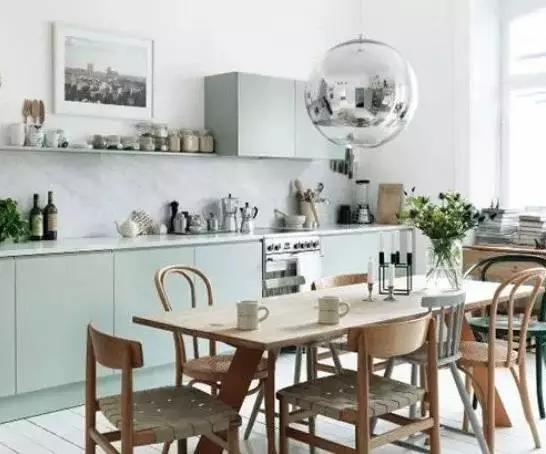▲客餐厅的布局第一步要确定的就是区分出“会客区”与“用餐区”的空间性质，再来进行细化布置，上图可以看到黄色区域是靠近厨房并且不会阻碍到通道的角落区域，可以设定为“用餐区”布置餐桌椅边，蓝色区域则是靠近阳台去一般设定为布置沙发的“会客区”。

下一步我们将区域功能细化，玄关没有靠墙区域可以布置鞋柜，我们还有一种方法就是用隔断柜的下柜了，可以布置的地方一是入门的左边，一是厨房门的右边，但后者更为好用，布置在这能化解入户门正对卧室门的风水忌讳，同时满足需求，所以我们将隔断鞋柜确定下来！

▲再来，用餐区要留过道将餐桌组合布置于靠墙区域，沙发处于阳台边上有更好的采光，相对的电视柜则是在沙发的对面，这样大致将平面的区域布置下来后再在立面上考虑它的柜体和家具摆设的功能性和美观度。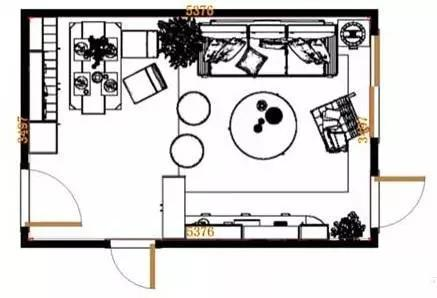▲这里比较需要思考的区域就是就餐区的利用，如果只是摆设普通的餐桌组合会空间不足且利用率偏低，所以用卡座的形式既能作为用餐的座位，整体的收纳功能也是十分强大。其他区域则是正常的布置形式即可。舌尖上的诱惑也许是清晨果蔬的原味，也许是结束了一天的工作回到家，吃上家人准备的热腾腾的饭菜。一个美妙的就餐环境更是必不可少。那么，什么样的餐厅能让你食欲大增？

1.如何考虑餐厅的界面处理？

所谓界面，是指形成一个使用空间所需要的地面、侧面和顶棚。餐厅的界面材料的品种、质地、色彩，与空间本身的特点有着密切的联系。

地面一般应选择表面光洁、易清洁的材料，如大理石、花岗岩、地砖。墙面在齐腰位置要考虑用些耐碰撞、耐磨损的材料，如选择一些木饰、墙砖，做局部装饰、护墙处理。顶棚宜以素雅、洁净材料做装饰，如乳胶漆、局部木饰，并用灯具作烘托，有时可适当降低顶棚，可给人以亲切感。

整个就餐空间，宜营造一种清新、优雅的氛围，以增添就餐者的食欲。若餐室内就餐空间太小时，则餐桌可以靠着有镜子的墙面摆放，或在墙角运用一些镜面与装饰，餐具柜相结合，可给人以宽敞感。

2.如何考虑餐厅的色彩布置？

总的说来，餐厅色彩宜以明朗轻快的色调为主。整体色彩搭配时，还应注意地面色调直深，墙面可用中间色调，天花板色调则浅，以增加稳重感。

在不同的时间、季节及心理状态下，人们对色彩的感受会有所变化，这时，可利用灯光来调节室内色彩气氛，以达到利于饮食的目的。家具颜色较深时，可通过明快清新的淡色或蓝白、绿白、红白相间的台布来衬托。

3.餐厅灯光应该如何布置？

除了伟大的演员，戏剧表演最重要的就是灯光照明，你的餐厅也不例外。通常使用光源集中照射餐桌、又不让整个房间过分明亮的暖色系照明往往有很好的效果。

餐厅非常适合使用吊灯。吊灯向下的光线，会形成一个光柱，会让一起吃饭的人有一种向心力，会诞生一个很温馨的氛围。只开吊灯感觉房间不够明亮的时候，可以将吊灯与间接照明并用以改善餐厅风味。

通常来说吊灯设置在距离餐桌75-90厘米大概是正常的高度。只要选好尺寸和挂在餐桌上方，不太可能不小心就撞到头。另外，要尽量选择抬头仰视时，光源不会伤害眼睛的灯具。使用延伸式餐桌时。可以通过安装配线导管，增设吊灯的方法来满足照明需要。

上面说了一个原则，就是不要房间有暗角。餐厅吊灯是向下的光柱，所以还需要其他环境光源来辅助。比如上面说的点阵光源是方法之一；壁灯也是非常好的方法；或者餐厅还能容纳得了餐边柜，放上一对小台灯也是非常好的方法，漂亮的烛台也可以。

还可以在餐具柜上放置台灯，提供与视线平行的照明。配有小巧灯罩的蜡烛灯台灯既不会占据大量的空间，散发的光线还会使你的肤色看起来更加迷人。

4.餐厅陈设应该如何布置？

餐厅的陈设既要美观，又要实用，不可信手拈来，随意堆砌。具体地讲，餐厅中的软装饰，如桌布、餐巾及窗帘等，应尽量选用较薄的化纤类材料，因为厚实的棉纺类织物，极易吸附食物气味且不易散去，不利于餐厅环境卫生；

花卉能起到调节心理、美化环境的作用，但切忌花花绿绿，使人烦燥而影响食欲。白色、粉色等淡色花会显得整个餐厅明亮活泼，可以促进食欲噢！

最重要的是，不要忘记装饰餐厅的墙壁。对家具较少的餐厅来说，墙壁上的装饰至关重要。在餐厅空无一物的墙壁上挂上一幅心仪的挂画或是照片是个很好的选择。

在长桌、餐具柜或桌子上面的空间也需要你用心装修。可以放置一个别致的花瓶器皿，需要注意的是，花瓶与餐桌的形式亦要和谐，长方形的餐桌，花瓶的插置宜构成三角形，而圆形餐桌，花瓶的插置以构成圆形为好。

其他的软装饰品，如字画、瓷盘、壁挂等，可根据餐厅的具体情况灵活安排，用以点缀环境，但要注意不可因此喧宾夺主，以免餐厅显得杂乱无章。

如果你正在攒钱来买你梦想的餐厅家具，不要剥夺同时使用这个房间的乐趣！你可以在二手家具市场选择一组价格不贵的折叠椅（需要搭配色彩活泼的软垫），再买一个大小合适的可折叠桌。一个有格调，让你食指大动的餐厅就诞生了，好好享用你的晚餐把！

你还应该知道这些行业秘密…

`声明：本文由入驻焦点开放平台的作者撰写，除焦点官方账号外，观点仅代表作者本人，不代表焦点立场错误信息举报电话： 400-099-0099，邮箱：jubao@vip.sohu.com，或点此进行意见反馈，或点此进行举报投诉。`A B C D E F G H J K L M N P Q R S T W X Y Z
A - B - C - D - E
• A
• 鞍山
• 安庆
• 安阳
• 安顺
• 安康
• 澳门
• B
• 北京
• 保定
• 包头
• 巴彦淖尔
• 本溪
• 蚌埠
• 亳州
• 滨州
• 北海
• 百色
• 巴中
• 毕节
• 保山
• 宝鸡
• 白银
• 巴州
• C
• 承德
• 沧州
• 长治
• 赤峰
• 朝阳
• 长春
• 常州
• 滁州
• 池州
• 长沙
• 常德
• 郴州
• 潮州
• 崇左
• 重庆
• 成都
• 楚雄
• 昌都
• 慈溪
• 常熟
• D
• 大同
• 大连
• 丹东
• 大庆
• 东营
• 德州
• 东莞
• 德阳
• 达州
• 大理
• 德宏
• 定西
• 儋州
• 东平
• E
• 鄂尔多斯
• 鄂州
• 恩施
F - G - H - I - J
• F
• 抚顺
• 阜新
• 阜阳
• 福州
• 抚州
• 佛山
• 防城港
• G
• 赣州
• 广州
• 桂林
• 贵港
• 广元
• 广安
• 贵阳
• 固原
• H
• 邯郸
• 衡水
• 呼和浩特
• 呼伦贝尔
• 葫芦岛
• 哈尔滨
• 黑河
• 淮安
• 杭州
• 湖州
• 合肥
• 淮南
• 淮北
• 黄山
• 菏泽
• 鹤壁
• 黄石
• 黄冈
• 衡阳
• 怀化
• 惠州
• 河源
• 贺州
• 河池
• 海口
• 红河
• 汉中
• 海东
• I
• J
• 晋中
• 锦州
• 吉林
• 鸡西
• 佳木斯
• 嘉兴
• 金华
• 景德镇
• 九江
• 吉安
• 济南
• 济宁
• 焦作
• 荆门
• 荆州
• 江门
• 揭阳
• 金昌
• 酒泉
• 嘉峪关
K - L - M - N - P
• K
• 开封
• 昆明
• 昆山
• L
• 廊坊
• 临汾
• 辽阳
• 连云港
• 丽水
• 六安
• 龙岩
• 莱芜
• 临沂
• 聊城
• 洛阳
• 漯河
• 娄底
• 柳州
• 来宾
• 泸州
• 乐山
• 六盘水
• 丽江
• 临沧
• 拉萨
• 林芝
• 兰州
• 陇南
• M
• 牡丹江
• 马鞍山
• 茂名
• 梅州
• 绵阳
• 眉山
• N
• 南京
• 南通
• 宁波
• 南平
• 宁德
• 南昌
• 南阳
• 南宁
• 内江
• 南充
• P
• 盘锦
• 莆田
• 平顶山
• 濮阳
• 攀枝花
• 普洱
• 平凉
Q - R - S - T - W
• Q
• 秦皇岛
• 齐齐哈尔
• 衢州
• 泉州
• 青岛
• 清远
• 钦州
• 黔南
• 曲靖
• 庆阳
• R
• 日照
• 日喀则
• S
• 石家庄
• 沈阳
• 双鸭山
• 绥化
• 上海
• 苏州
• 宿迁
• 绍兴
• 宿州
• 三明
• 上饶
• 三门峡
• 商丘
• 十堰
• 随州
• 邵阳
• 韶关
• 深圳
• 汕头
• 汕尾
• 三亚
• 三沙
• 遂宁
• 山南
• 商洛
• 石嘴山
• T
• 天津
• 唐山
• 太原
• 通辽
• 铁岭
• 泰州
• 台州
• 铜陵
• 泰安
• 铜仁
• 铜川
• 天水
• 天门
• W
• 乌海
• 乌兰察布
• 无锡
• 温州
• 芜湖
• 潍坊
• 威海
• 武汉
• 梧州
• 渭南
• 武威
• 吴忠
• 乌鲁木齐
X - Y - Z
• X
• 邢台
• 徐州
• 宣城
• 厦门
• 新乡
• 许昌
• 信阳
• 襄阳
• 孝感
• 咸宁
• 湘潭
• 湘西
• 西双版纳
• 西安
• 咸阳
• 西宁
• 仙桃
• 西昌
• Y
• 运城
• 营口
• 盐城
• 扬州
• 鹰潭
• 宜春
• 烟台
• 宜昌
• 岳阳
• 益阳
• 永州
• 阳江
• 云浮
• 玉林
• 宜宾
• 雅安
• 玉溪
• 延安
• 榆林
• 银川
• Z
• 张家口
• 镇江
• 舟山
• 漳州
• 淄博
• 枣庄
• 郑州
• 周口
• 驻马店
• 株洲
• 张家界
• 珠海
• 湛江
• 肇庆
• 中山
• 自贡
• 资阳
• 遵义
• 昭通
• 张掖
• 中卫

1室1厅1厨1卫1阳台

1
2
3
4
5

0
1
2

1

1

0
1
2
3报名成功，资料已提交审核A B C D E F G H J K L M N P Q R S T W X Y Z
A - B - C - D - E
• A
• 鞍山
• 安庆
• 安阳
• 安顺
• 安康
• 澳门
• B
• 北京
• 保定
• 包头
• 巴彦淖尔
• 本溪
• 蚌埠
• 亳州
• 滨州
• 北海
• 百色
• 巴中
• 毕节
• 保山
• 宝鸡
• 白银
• 巴州
• C
• 承德
• 沧州
• 长治
• 赤峰
• 朝阳
• 长春
• 常州
• 滁州
• 池州
• 长沙
• 常德
• 郴州
• 潮州
• 崇左
• 重庆
• 成都
• 楚雄
• 昌都
• 慈溪
• 常熟
• D
• 大同
• 大连
• 丹东
• 大庆
• 东营
• 德州
• 东莞
• 德阳
• 达州
• 大理
• 德宏
• 定西
• 儋州
• 东平
• E
• 鄂尔多斯
• 鄂州
• 恩施
F - G - H - I - J
• F
• 抚顺
• 阜新
• 阜阳
• 福州
• 抚州
• 佛山
• 防城港
• G
• 赣州
• 广州
• 桂林
• 贵港
• 广元
• 广安
• 贵阳
• 固原
• H
• 邯郸
• 衡水
• 呼和浩特
• 呼伦贝尔
• 葫芦岛
• 哈尔滨
• 黑河
• 淮安
• 杭州
• 湖州
• 合肥
• 淮南
• 淮北
• 黄山
• 菏泽
• 鹤壁
• 黄石
• 黄冈
• 衡阳
• 怀化
• 惠州
• 河源
• 贺州
• 河池
• 海口
• 红河
• 汉中
• 海东
• I
• J
• 晋中
• 锦州
• 吉林
• 鸡西
• 佳木斯
• 嘉兴
• 金华
• 景德镇
• 九江
• 吉安
• 济南
• 济宁
• 焦作
• 荆门
• 荆州
• 江门
• 揭阳
• 金昌
• 酒泉
• 嘉峪关
K - L - M - N - P
• K
• 开封
• 昆明
• 昆山
• L
• 廊坊
• 临汾
• 辽阳
• 连云港
• 丽水
• 六安
• 龙岩
• 莱芜
• 临沂
• 聊城
• 洛阳
• 漯河
• 娄底
• 柳州
• 来宾
• 泸州
• 乐山
• 六盘水
• 丽江
• 临沧
• 拉萨
• 林芝
• 兰州
• 陇南
• M
• 牡丹江
• 马鞍山
• 茂名
• 梅州
• 绵阳
• 眉山
• N
• 南京
• 南通
• 宁波
• 南平
• 宁德
• 南昌
• 南阳
• 南宁
• 内江
• 南充
• P
• 盘锦
• 莆田
• 平顶山
• 濮阳
• 攀枝花
• 普洱
• 平凉
Q - R - S - T - W
• Q
• 秦皇岛
• 齐齐哈尔
• 衢州
• 泉州
• 青岛
• 清远
• 钦州
• 黔南
• 曲靖
• 庆阳
• R
• 日照
• 日喀则
• S
• 石家庄
• 沈阳
• 双鸭山
• 绥化
• 上海
• 苏州
• 宿迁
• 绍兴
• 宿州
• 三明
• 上饶
• 三门峡
• 商丘
• 十堰
• 随州
• 邵阳
• 韶关
• 深圳
• 汕头
• 汕尾
• 三亚
• 三沙
• 遂宁
• 山南
• 商洛
• 石嘴山
• T
• 天津
• 唐山
• 太原
• 通辽
• 铁岭
• 泰州
• 台州
• 铜陵
• 泰安
• 铜仁
• 铜川
• 天水
• 天门
• W
• 乌海
• 乌兰察布
• 无锡
• 温州
• 芜湖
• 潍坊
• 威海
• 武汉
• 梧州
• 渭南
• 武威
• 吴忠
• 乌鲁木齐
X - Y - Z
• X
• 邢台
• 徐州
• 宣城
• 厦门
• 新乡
• 许昌
• 信阳
• 襄阳
• 孝感
• 咸宁
• 湘潭
• 湘西
• 西双版纳
• 西安
• 咸阳
• 西宁
• 仙桃
• 西昌
• Y
• 运城
• 营口
• 盐城
• 扬州
• 鹰潭
• 宜春
• 烟台
• 宜昌
• 岳阳
• 益阳
• 永州
• 阳江
• 云浮
• 玉林
• 宜宾
• 雅安
• 玉溪
• 延安
• 榆林
• 银川
• Z
• 张家口
• 镇江
• 舟山
• 漳州
• 淄博
• 枣庄
• 郑州
• 周口
• 驻马店
• 株洲
• 张家界
• 珠海
• 湛江
• 肇庆
• 中山
• 自贡
• 资阳
• 遵义
• 昭通
• 张掖
• 中卫• 手机• 分享
• 设计
免费设计
• 计算器
装修计算器
• 入驻
合作入驻
• 联系
联系我们
• 置顶
返回顶部